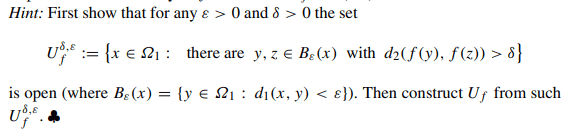# Basic Measure Theory: Borel ##\sigma##- algebra

• I
• WMDhamnekar

#### WMDhamnekar

MHB
TL;DR Summary
Let ##(\Omega_1, d_1)## and ##(\Omega_2, d_2)## be metric spaces and let ##f : \Omega_1 \to \Omega_2 ## be an arbitrary map. Denote by ##U_f =\{x \in \Omega_1 :## f is discontinuous at ##x\}## the set of points of discontinuity of ##f##. Show that ##U_f \in \mathcal{B}(\Omega_1).##We can proceed as follows. Let ##x \in U_f##. Since ##f## is discontinuous at ##x##, there exists ##\varepsilon > 0## such that for some ##\delta > 0##, we can find ##y, z \in B_{\varepsilon}(x)## with ##d_2(f(y),f(z)) > \delta##. Therefore, ##x \in U^{\delta,\varepsilon}_f##. So, ##U_f \subset U^{\delta,\varepsilon}_f##. Since ##U^{\delta,\varepsilon}_f## is open, any subset of it is also open. So ##U_f## is open.

Next, we show that for any ##\delta, \varepsilon>0##, we have ##U^{\delta, \varepsilon}_f \subset U_f##. Let ##x \in U^{\delta, \varepsilon}_f##. Then there exist ##y,z \in B_{\varepsilon}(x)## such that ##d_2(f(y),f(z)) > \delta##. Since ##f(y) \neq f(z)##, ##f## cannot be continuous at ##x##. Therefore, ##x \in U_f##. Thus, ## U^{\delta,\varepsilon}_f \subset U_f##.

Taking intersection over all ##\delta##, ##\varepsilon##, we have ##U_f = \bigcap_{ \delta, \varepsilon > 0}U^{\delta,\varepsilon}_f##. Since intersections of open sets are open, ##U_f## is open.
Here is an example:

Let ##\Omega_1 = \mathbb{R}## with the usual metric ##d_1(x,y) = |x-y|##, and ##\Omega_2 = \mathbb{R}^2## with the Euclidean metric ##d_2((x_1,y_1),(x_2,y_2)) = \sqrt{(x_1-x_2)^2 + (y_1-y_2)^2}##.

Define ##f:\mathbb{R} \to \mathbb{R}^2## as ##f(x) = (x, x^2)## if ##x \neq 0## and ##f(0) = (0,1)##.

Then, ##f## is discontinuous at ##x=0##. For any ##\varepsilon > 0##, taking ##\delta = 1##, we can find ##y = -\varepsilon/2 < 0## and ##z = \varepsilon/2 > 0## in ##B_{\varepsilon}(0)## such that ##d_2(f(y),f(z)) = |(-1/2,0) - (1/2,0)| = 1 > \delta = 1##. Therefore, ##0 \in U^{\delta,\varepsilon}_f## for all ##\delta, \varepsilon > 0##. Hence, ##0 \in U_f##.

Also, for any ##x \neq 0, f## is continuous at ##x##. Therefore, ##U_f = \{0\}##. Since ##\{0\}## is open in ##\mathbb{R}##, ##U_f## is open.

Hence, in this example, ##U_f = \{0\} \in \mathcal{B}(\Omega_1 = \mathbb{R})##.

Therefore, ##U_f## is an open subset of ##\Omega_1##. Hence, ##U_f \in \mathcal{B}(\Omega_1)##.

Is this answer and example correct?

Last edited: